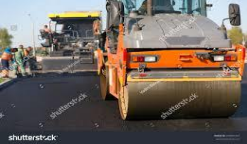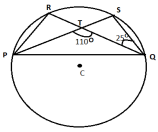Subject: Maths, asked 3 hours, 43 minutes ago

Pls factorise thisSubject: Maths, asked 4 hours, 6 minutes ago

IN A CIRCLE WITH CENTRE O, QRS = 40º what is the value of PQR

Subject: Maths, asked 4 hours, 19 minutes ago

THE ANGLE BETWEEN THE TWO ALTITUDES OF A PARALLELOGRAM EFGH THROUGH THE VERTEX H WHICH IS AN OBTUSE ANGLE OF THE PARALLELOGRAM IS 85º WHAT IS THE MEASURE OF EACH ANGLE OF THE PARALLELOGRAM

Subject: Maths, asked 4 hours, 29 minutes ago

triangle xyz is a triangle right angled at y and m is the midpoint of XZ if XZ is 17cm then find MY

Subject: Maths, asked 4 hours, 36 minutes ago

pqrs is a rhombus with rsp=109º what is the value of rpq

Subject: Maths, asked 5 hours ago

WHAT is the degree of the polynomial (x^2-4x) (x^2-5x) -24

Subject: Maths, asked 12 hours, 52 minutes ago

Degree.of the polynomial,

Subject: Maths, asked 14 hours, 44 minutes ago

A copper wire of diameter 0.1 mm is made from a copper bar of volume 1cm3 . Find the length of the wire.

Subject: Maths, asked 14 hours, 59 minutes ago

6. Prove that the line joining the mid points of the two parallel chords on the both  sides of the centre of a circle passes through the centre. (4) 7. Some PWD workers were constructing a road using a road roller. The 1 m long road  roller is made of iron. Its inner diameter was 54 cm and the thickness was 9cm.(i) Find the outer curved surface area of the road roller. (2) (ii) If they can construct 1584 m2road in a day, find the revolutions that the road  roller made. (2)

Subject: Maths, asked 15 hours agoIn the given figure find the value of <PRQ.

Subject: Maths, asked 15 hours, 12 minutes ago

Find the degree of the polynomial. Expert pls answer fast. Thank you

Subject: Maths, asked 1 day, 2 hours ago

Ruchika and her friends went on a trip. She slept in a conical tent. They discovered that the diameter of their conical tent is 4.2 m. One of her friends observed the tent's packing, which stated that the volume of the tent is 12.936 m^3 and it's height is 2.8 m and slant height is 3.5 m, which piqued the children's interest in learning more about the conical tent they were carrying. Based on the above information, answer the following question: What would be the curved surface area of the conical tent?

Subject: Maths, asked 1 day, 3 hours ago

Pls help me pls pls

Subject: Maths, asked 1 day, 3 hours ago

Pls tell 41

Subject: Maths, asked 1 day, 3 hours ago

Pls tell anyone

What are you looking for?